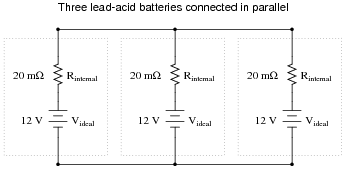# Batteries

## Basic Electricity

• #### Question 1

If I wanted to build a battery-powered electric drill, would it be better to use primary cell batteries, or secondary cell batteries? Explain your answer.

• #### Question 2

Identify some common “primary” cell types, as well as some common “secondary” cell types. For each of these battery types, identify their standard output voltages.

• #### Question 3

Batteries store energy in the form of chemical bonds between atoms. When a complete circuit is connected to the terminals of a battery, a current begins inside the battery and through the circuit. Chemical reactions begin inside the battery at the same time the circuit is formed, fueling this current. When all the chemicals have been depleted, though, the current will cease. This is what happens when a battery “dies.”

Explain what takes place when a battery is recharged, and what electrical device(s) must be connected to a battery to recharge it. Be as specific as possible in your answer.

• #### Question 4

Draw a schematic diagram showing a DC generator charging a battery, and another schematic showing a battery discharging into a resistive load. Be sure to include arrows in your drawing showing the direction of charging current.

• #### Question 5

What does the amp-hour capacity of a chemical battery represent? What does it mean if a battery is rated at, say, 150 amp-hours?

• #### Question 6

What is the best-performing secondary cell technology today, in terms of energy stored per unit mass?

• #### Question 7

Suppose I desired to power the light bulbs in a remote cabin with solar power, charging a large battery bank. The battery bank needs to be able to power ten 60-watt light bulbs for eight hours without being recharged. The system voltage is 115 volts. How large must the battery bank be, in total amp-hours?

• #### Question 8

Suppose a 12 volt lead-acid battery has an internal resistance of 20 milli-ohms (20 mΩ):If a short-circuit were placed across the terminals of this large battery, the fault current would be quite large: 600 amps!

Now suppose three of these batteries were connected directly in parallel with one another:Reduce this network of parallel-connected batteries into either a Thévenin or a Norton equivalent circuit, and then re-calculate the fault current available at the terminals of the three-battery “bank” in the event of a direct short-circuit.

• #### Question 9

This heavy-duty battery test circuit seems to have a problem. Instead of the voltmeter drooping when the test switch is closed, it remains steady regardless of switch state. This happens even when the battery under test is known to be weak:Other clues to the problem include the fact that rheostat setting seems to make no difference in the voltmeter’s indication, and also that the cooling fan motor does not come on when the switch is pressed.

Identify possible faults in this circuit that could account for the symptoms listed here. Also, explain why each of your proposed faults would cause the observed symptoms.

• #### Question 10

Some brands of dry-cell batteries come equipped with a built-in charge indicator in the form of a thermal strip along one side. Pressing two white dots closes a circuit, which heats up the strip and indicates battery charge by changing color.

Compare the accuracy of a charge indicator of this general design against using a voltmeter to measure open-circuit battery voltage. Which method of measurement is a more accurate indication of battery charge, and why?

• #### Question 11

What does it mean if a battery, or a bank of batteries, receives a floating charge? What does it mean if a battery, or a bank of batteries, receives an equalizing charge?

• #### Question 12

An important equation in predicting the capacity of a lead-acid cell is called Peukert’s formula:

 In t = C

Where,

I = Discharge current, in amps

n = Constant, particular to the cell (typically between 1.1 and 1.8)

t = Time of discharge

C = Constant, particular to the battery (varies with the amp-hour capacity)

Explain how Peukert’s formula relates to the simpler “amp-hour” formula.

Also, calculate the discharge time for a battery with an n value of 1.2 and a C value of 200, given a discharge current of 25 amps.

• #### Question 13

A battery has an advertised capacity of 600 amp-hours, taken from tests performed over an 8-hour period (where capacity in amp-hours = It). According to Peukert’s formula, what rate of current discharge will result in the battery fully discharging in 3 hours (assume an n value of 1.35)?